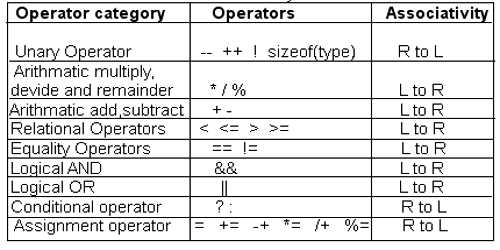The term unary defines operators in binary algebra, trinary algebra, arithmetic, and set theory. Sometimes a unary operation is called a monadic operation or a singulary operation. Unary operations only involve one quantity such as negation, absolute value or trigonometric functions.

In set theory, a unary operation on set A is a function A → A.

More generally, a unary operation is a function with exactly one operand, such as the factorial, square root, or NOT.

Common notations are prefix notation (e.g. +, −, not), postfix notation (e.g. factorial n!), functional notation (e.g. sin x or sin(x)), and superscripts (e.g. transpose AT). Other notations exist as well. For example, in the case of the square root, a horizontal bar extending the square root sign over the argument can indicate the extent of the argument.Unary negative and positive

As unary operations have only one operand they are evaluated before other operations containing them in common mathematics (because certain programming languages do not abide by such rules). Here is an example using negation:

These two unary operations, ‘+’ and ‘-‘, have precedence over all binary operations. To see this, let’s look at a situation which is often termed a ‘double negative’ or stated as ‘two negatives make a positive’. Consider this expression:

3 − −2

In this expression there are two operators that look like this: ‘-‘. They are not the same. The first one, (left most), is the binary subtraction operator. The other, (right before the 2), is the unary negative sign operator. Here the first ‘−’ represents the binary subtraction operation, while the second ‘−’ represents the unary negation of the 2 (or ‘−2’ could be taken to mean the integer −2). Therefore, the expression is equal to:

3 − (−2) = 5

Technically there is also a unary positive but it is not needed since we assume a value to be positive:

(+2) = 2

Unary positive does not change the sign of a negative operation:

(+(−2)) = (−2)

In this case a unary negative is needed to change the sign:

(−(−2)) = (+2)

Information Source: